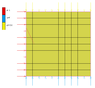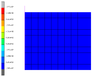# Poisson Ratio -- Finding a corresponding analytical solution for the strain

• Br--1995
In summary, the conversation discusses the use of the poisson ratio in a FE simulation and the discrepancies between the analytical solution and the simulation results. The analytical solution for strain_xx is derived and it is found to be equal to -v * strain_yy / (1-v) = 0.0485714... in plain strain situation. The conversation also mentions the relation between stress and strain in the x-direction and the importance of considering the freedom of expansion in the right x-direction.

#### Br--1995

TL;DR Summary
Find a corresponding analytical solution for the strain in the x-direction caused by the poisson ratio.
Hi, I ran into problems using the poisson ratio.
For a FE simulation I created a simple 2D 1mm x 1mm block, and prescribed a 0.1 mm displacement at the top edge.
Furthermore, the bottom edge is constraint in the y-dir, and the left edge in the x-dir.
The material parameters are E = 100, and v (poisson ratio) = 0.3.
Note the simulation is executed for a plane strain assumption!

To verify the results I would like to solve the analytical solution for this problem.
This is quite simple tbh, I use the 2D strain-stress relations for the plane strain problem.
However, the simulation shows that the strain_yy = -0.1 (as expected) but the strain_xx = 0.04285714.

I really cannot figure out the analytical solution for the strain_xx, I would expect this to be 0.03 (strain_xx = -v * strain_yy).

I know that the 0.0428.. value should be the correct one, because I tried the simulation in different simulation software.
Hopefully someone can explain me how to get this value analytically?Last edited by a moderator:
Just by looking at the numbers: strain_xx = -v * strain_yy / (1-v) = 0.0485714...

•Br--1995
In the plain strain situation, the strain in the 3rd direction is zero. This results in a larger strain in the transverse direction. Try the calculation with plain stress and see what you get. For plain strain, you get $$\epsilon_y=-\frac{\nu}{(1-\nu)}\epsilon_x=-0.04286$$

Last edited:
•Br--1995
Chestermiller said:
In the plain strain situation, the strain in the 3rd direction is zero. This results in a larger strain in the transverse direction. Try the calculation with plain stress and see what you get. For plain strain, you get $$\epsilon_y=-\frac{\nu}{(1-\nu)}\epsilon_x=-0.04286$$
Thank you very much for your answer, that's the relation I was looking for. (Note, there is a small typo: $$\epsilon_x$$ and $$\epsilon_y$$ should be the other way around)

To make the story complete, I expect this relation is derived by:
$$\sigma_x = \frac{E}{(1+\nu)(1-2\nu)} [(1-\nu) \epsilon_x + \nu \epsilon_y]$$

Because the block is not constraint in the right x-dir, it can expand freely -> conclusion: $$\sigma_x = 0$$
Therefore, we can write the previous eq. for the strain in the x-dir as :
$$\epsilon_x = -\frac{\nu}{1-\nu} \epsilon_y$$

Correct me if I am wrong.

Br--1995 said:
Thank you very much for your answer, that's the relation I was looking for. (Note, there is a small typo: $$\epsilon_x$$ and $$\epsilon_y$$ should be the other way around)

To make the story complete, I expect this relation is derived by:
$$\sigma_x = \frac{E}{(1+\nu)(1-2\nu)} [(1-\nu) \epsilon_x + \nu \epsilon_y]$$

Because the block is not constraint in the right x-dir, it can expand freely -> conclusion: $$\sigma_x = 0$$
Therefore, we can write the previous eq. for the strain in the x-dir as :
$$\epsilon_x = -\frac{\nu}{1-\nu} \epsilon_y$$

Correct me if I am wrong.
That's not exactly the way I did it, but it is correct. I hope you also noticed that ##\sigma_y## is not equal to ##E\epsilon_y##, but rather $$\frac{E\epsilon_y}{(1-\nu^2)}$$

•Br--1995
Chestermiller said:
That's not exactly the way I did it, but it is correct. I hope you also noticed that ##\sigma_y## is not equal to ##E\epsilon_y##, but rather $$\frac{E\epsilon_y}{(1-\nu^2)}$$
Thank you for your help! Yes I've noticed this.

## 1. What is the Poisson ratio and how is it related to strain?

The Poisson ratio is a measure of the ratio of lateral strain to axial strain in a material. It is denoted by the Greek letter ν and is mathematically defined as the negative of the ratio of transverse strain to axial strain. In other words, it quantifies how much a material will expand or contract in one direction when it is stretched or compressed in another direction.

## 2. How is the Poisson ratio calculated?

The Poisson ratio is typically calculated using the following formula: ν = -εtl, where εt is the transverse strain and εl is the axial strain. This can also be expressed as ν = -(Δw/w)/(Δl/l), where Δw is the change in width and Δl is the change in length.

## 3. What is an analytical solution for finding the strain?

An analytical solution for finding the strain refers to a mathematical or theoretical approach to solving for the strain in a material. This involves using equations and mathematical models to determine the strain, rather than relying on experimental or empirical data.

## 4. How do you find a corresponding analytical solution for the strain?

To find a corresponding analytical solution for the strain, one must first determine the material properties and boundary conditions, such as the applied load and geometry of the material. Then, mathematical equations and models can be used to solve for the strain. This often involves using differential equations and boundary value problems.

## 5. What are some common applications of the Poisson ratio and strain analysis?

The Poisson ratio and strain analysis have many practical applications in various fields, including engineering, materials science, and geology. They are used to predict how materials will behave under stress, to design and test structures, and to study the properties of different materials. They are also important in understanding the behavior of rocks and soils, and in medical imaging techniques such as MRI.